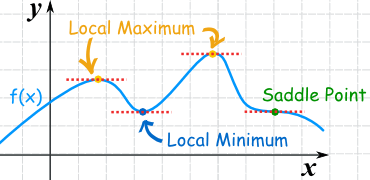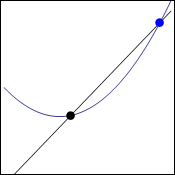# Derivatives made easy 3a) Maximum and minimum explained

Hi,
in school we learn how to derive right now. The teacher does not do very good job. The class does not know about limits and they only learned power rule and no other rule for derivation though at the same time they learn about maxima and minima.

When you derive function you can find lot of useful informations about it. Today I will talk about minima and maxima. Those are points where the function turns around creates “hill” or “valley” – maxima or minima.Some fictional function.

In the function above (f-blue line) you can see couple of points marked. Two orange points are maxima, blue is minima and green is special to which I will get in another post.

As I have already said quite few times, when you derive function you will find out what is the slope (how much up or down) at one point. If it does not go up or down it is zero, those are the maxima and minima you are looking for. Right before maximum you have positive slope, the function goes up towards maximum. Right after maximum you have negative slope. What is between if the function is smooth? Zero. When we derive function, lets say function like this:

f(x)=2x²    We get (according to power rule, if you do not know it ignore it right now):

f'(x)=4x    The apostrophe means that it has been derived.

Now when does this derivation equals zero? In other words? When is the slope zero?

Only when x=0 because f'(0)=4*0 = 0 

The original function has minimum or maximum on the point when x=0 . Tricky is that you do not know if it is minimum or maximum which needs some more work and I will get to it in another post.

Dragallur

I will also talk about power rule, multiplication rule and so on in the future.

To get the coordinates of the maximum or minimum you just plug the solution of the derivative to original function and you will get the y coordinate.

If you work with higher order polynomials like x³ you might easily get more solutions which means more minimas and maximas.

If you plot the derivation you will get the slope anywhere.

PS: sometimes I see minima or minimum and maxima or maximum so I use both as I want.

# Jerks are even in physics

Hi,
the title is a pun. There are probably jerks yes, but what I want to talk about is physical unit called jerk, it is named like this because jerk not only means, idiot or stupid, but also to move suddenly, because of surprise.

It will be nice, if we first recall that derivation describes the rate of change of something. For example, speed is the first derivative of position because speed describes the rate of change of position, the higher the speed the more position changes!1st derivation of position “compared” to time

In the picture above you can see how speed is defined compared to position (x) and time (t). It is its derivative as I said before. Now of course you can define something, that describes how velocity changes over time. That is called acceleration.Again, “d” simply means derivation and when it is “squared” it means that you need to derive it twice. Acceleration describes, how velocity changes over time.

This is all you might need for daily life. Of course though, scientists defined next derivations, the change of acceleration in time is called jerk. The change of jerk is called jounce the change of jounce is crackle, next follows pop and then possibly lock, drop, shot and put. The SI units of all of these time, position related things are similar. With each derivation you add one to exponent of time.

v=m/s¹
a=m/s²
j=m/s³

and so on..

Just to remind you, if you have lets say “pop”, which is 6th derivation, of 10 m/s^6 you will have a tremendous speed extremely fast. From this next equation it should be pretty clear:The power of the higher derivations is that the exponent does extreme changes in a moment. r is the position, v speed, a acceleration… p is pop and t is time.

This is probably not used much, if at all, even in engineering.. but hey, fun!

Dragallur

It is not a unit. It is physical quantity or something like that. I do not really know how it is called in english.

# Quick point about equations and graphs

Hi,
mliae asked me to make some simpler post so she understands this post. So here it comes, she said that it was long since she used equations:

Well equation is something like this:

x=1

That is quite clear. Of course you can have very complicated equation with many “unknowns” which are usually noted as letters, x for example. All thats easy and it says that something (x) is equal to one, it has the value of 1.

We can manipulate these equations if we abide one rule: both sides (from left and right of equal sign) have to be manipulated. If we add one we have to do it on both sides:

x+1=1+1     —>    x+1=2

Easy. We can substitute in equations if we work with more:

a=b+42
2a+3=x

2(b+42)+3=x    (“a” was substitued by b+42 because that is what it equals to.)

Now in the post that is our concern we used this equation:To get here we have to use graph but I did not stop on that very much so I will go through it again.

Points on graph have two coordinates. This is because graph has 2 dimensions. These coordinates are usually called x and y and they are noted like this:

(x;y)

x says how much the point is to the left or right and y says how up or down (or closer/ further)

We had two points there on the graph:Now we will take the blue point as stacionary of course but since we are working in general and not with specific numbers, it should not matter.

So black point has coordinates x and y (x;y)

Since we want it to be general we will left it like this except the y. This is because we are going to derive functions and in those y=f(x) which means that y coordinate of the point is f(x). That is the notation that is used. We are working with the function f that gave coordinate y to our black point.

black_point(x;f(x))
blue_point(x+h;f(x+h))

The h should be clear from last time. It is the distance from the black point. Then when you insert this into the “slope” equation which I talked about here, you will get what you want.

Dragallur

Feel free to ask for more clarification.

# Derivatives made easy 1) Slope on curve

Hi,
today I am going to explain one fundamental equation that is used to calculate derivatives.

Derivative of a function basically says what is the rate of change or the slope of that function. You can have both rate of change of whole function which gives you another function or just at one point which equals to some number. The fundamental thing is to find the slope. Last time I explained this only for a line, not curve. On line the slope is constant but not on curve.Tangent and secant line.

On the gif above you can see a function. There is a black point that is on the same place. Blue point is getting closer and closer with each step. All the time the black line creates secant line to the curve, it cuts it on two points. When it is red it is tangent line which means that it does not cuts it at all. It is the only one step in which it is just touching. To get the rate of change of curve you need to find this tangent line. Lets call the distance between the two points “h”. The tangent line will exist when h approaches zero.THE equation. Sometimes f'(x) is written on the left.

This thing above is the equation that we will get when we take coordinates of both points.

black point(  x;f(x)   )                    
blue point  (  x+h;f(x+h)  )           

Just to clear things out, the first thing in the brackets is the x coordinate and the second y coordinate. As I said blue point is moved to side by the distance h and as the distance approaches 0 we are getting tangent line of curve. In the last post I showed that you need to only divide the difference of y coordinates by difference of x coordinates. Check it out if you do not know what I mean.

We will do exactly it to get right part of the equation above without the limit. Only h will be left in the denominator because there is the difference in x coordinates which means:

x+h-x=h

Now to get the tangent line we only add the limit and we have got the fundamental equation of derivates. This we can use for any curve or line that we want to derive. The left part is just a notation for derivation.

Dragallur

PS: be sure to check out this page that lets you interactively create secant and tangent lines.

Just in case you do not understand why there is f(), it is basicly the same as y though we are in functions so we use this kind of notation and yes, f(x)=y. We just add h to get the y coordinate of blue point.